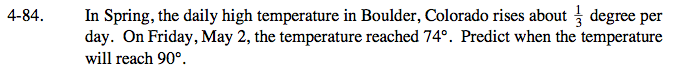### Home > CAAC > Chapter 4 > Lesson 4.2.2 > Problem4-84

4-84.Using the equation y = mx + b, put the word problem into equation form.

What is the growth rate?

What is the starting point?

What is the desired temperature?

Now, solve for x.

$\frac{1}{3}x$

$\frac{1}{3}x + 74$

$90=\frac{1}{3}x + 74$

48 days later
(Thursday, June 19).